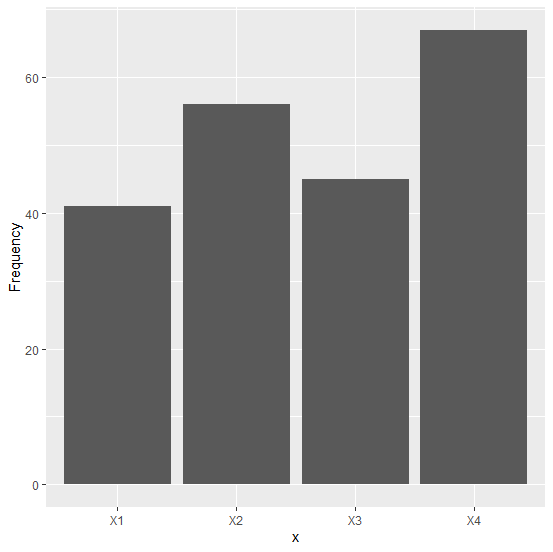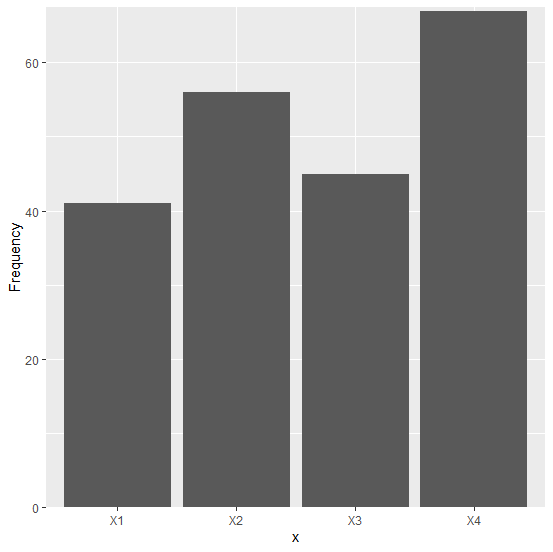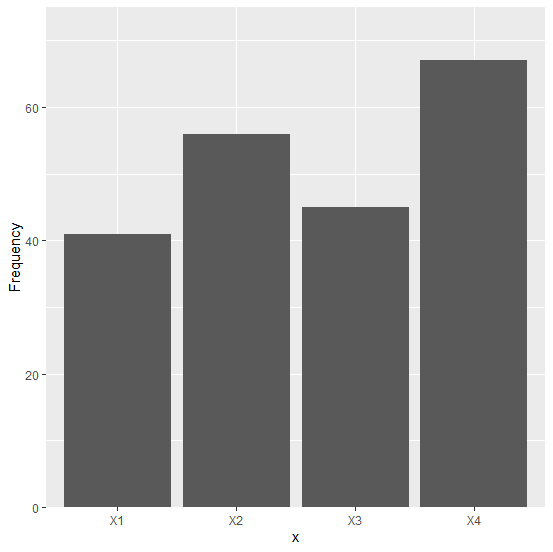# How to manage top and bottom spaces of a bar plot using ggplot2 in R?

A bar plot is one of the most commonly used plots for categorical data and it can be easily done in R with the help of ggplot2. When we create a bar plot using ggplot2, there exists some space between bars and the X-axis and the largest bar and top area of the plot. This can be reduced or increased by using scale_y_continuous function.

## Example

Consider the below data frame −

x <-c("X1","X2","X3","X4")
Frequency <-c(41,56,45,67)
df<-data.frame(x,Frequency)
library(ggplot2)

Creating a simple bar plot −

ggplot(df,aes(x,Frequency))+geom_bar(stat="identity")

## OutputCreating a simple bar plot with zero space between bars and X-axis and a decreased top area −

## Example

ggplot(df,aes(x,Frequency))+geom_bar(stat="identity")+
+ scale_y_continuous(expand=c(0,0),limits=c(0,67.5))

## OutputCreating a simple bar plot with zero space between bars and X-axis and an increased top area −

## Example

ggplot(df,aes(x,Frequency))+geom_bar(stat="identity")+
+ scale_y_continuous(expand=c(0,0),limits=c(0,75))

## Output